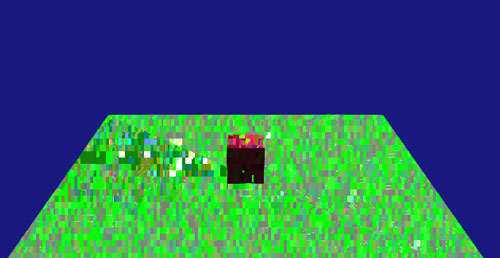# Flickering artefacts - coloured squares

Hi !
First, sorry for my bad English.
Recently, I reinstall my computer, and now my very simple shaders give me strange flickering artefacts in form of coloured squares.Before re-installation, I didn’t have those artefacts. If I remove the code portion that bind to shader and set its Uniforms, I have no problems.
Here are my shaders:

``````#version 120
varying vec3 lightVec;
varying vec3 normal;

uniform vec3 lightPosition;
uniform float lightIntensity;
uniform int lightOn;

void main(void)
{
gl_Position = ftransform();
normal = normalize(gl_NormalMatrix * gl_Normal);
lightVec = normalize(gl_NormalMatrix * lightPosition);
gl_FrontColor = gl_Color;
gl_TexCoord = gl_TextureMatrix * gl_MultiTexCoord0;
}
``````

``````#version 120
varying vec3 lightVec;
varying vec3 normal;

uniform float lightIntensity;
uniform int lightOn;
uniform sampler2D tex;

void main(void)
{
float intensity;
vec4 texel;
vec3 baseColor;
vec3 fragColor;

baseColor = gl_Color.rgb;

texel = texture2D(tex,gl_TexCoord.st);
baseColor *= texel.rgb;

intensity = max(dot(lightVec,normalize(normal)),0.0) * lightIntensity;

fragColor += baseColor;
fragColor += (gl_FrontMaterial.diffuse).rgb;
fragColor += (gl_FrontMaterial.ambient).rgb;

if(lightOn >= 1)
{
fragColor *= intensity;
}

gl_FragColor = vec4(fragColor,1.0);
}
``````

Is it a common kind of artefacts when beginning GLSL ? Is it really a mistake from my part in shaders ? Or could it be a problem in uniforms settings in my program ?